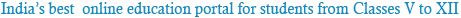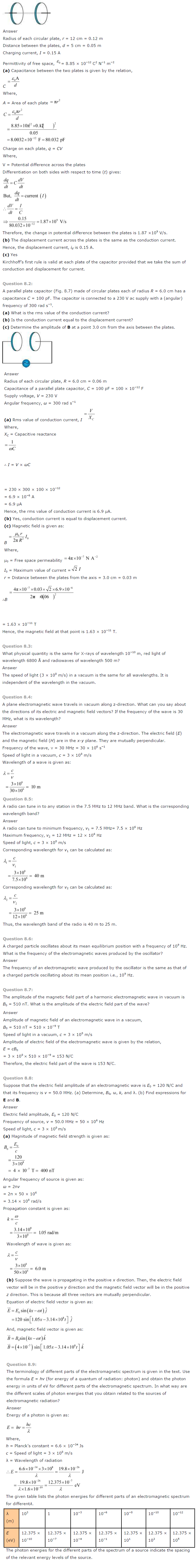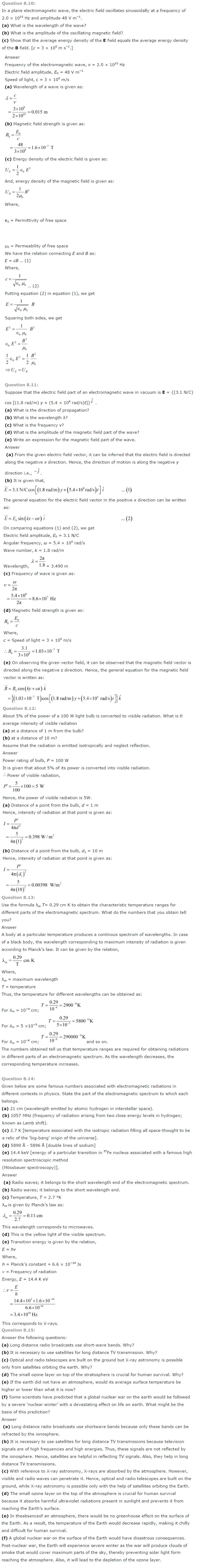### NCERT Solutions for Class 12th Physics Chapter 8: Electromagnetic Waves

Question 8.1:
Figure 8.6 shows a capacitor made of two circular plates each of radius 12 cm, and separated by 5.0 cm. The capacitor is being charged by an external source (not shown in the figure). The charging current is constant and equal to 0.15 A.
(a) Calculate the capacitance and the rate of charge of potential difference between the plates.
(b) Obtain the displacement current across the plates.
(c) Is Kirchhoff’s first rule (junction rule) valid at each plate of the capacitor? Explain.Powered by: iVerticals Web Technology Pvt Ltd